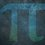# UKMT Special (Problem $1$)

Show that there are at least $3$ primes that are less than $200$ that satisfy the system of equations:

$p + 2$

$p + 6$

$p + 8$

$p + 12$

and result in primes.

Also show that there is only one one prime that satisfy the system of equations:

$q + 2$

$q + 6$

$q + 8$

$q + 12$

$q + 14$

and result in primes.

[UKMT BMO $2019$, Q$1$]Note by Yajat Shamji
8 months, 1 week ago

This discussion board is a place to discuss our Daily Challenges and the math and science related to those challenges. Explanations are more than just a solution — they should explain the steps and thinking strategies that you used to obtain the solution. Comments should further the discussion of math and science.

When posting on Brilliant:

• Use the emojis to react to an explanation, whether you're congratulating a job well done , or just really confused .
• Ask specific questions about the challenge or the steps in somebody's explanation. Well-posed questions can add a lot to the discussion, but posting "I don't understand!" doesn't help anyone.
• Try to contribute something new to the discussion, whether it is an extension, generalization or other idea related to the challenge.

MarkdownAppears as
*italics* or _italics_ italics
**bold** or __bold__ bold
- bulleted- list
• bulleted
• list
1. numbered2. list
1. numbered
2. list
Note: you must add a full line of space before and after lists for them to show up correctly
paragraph 1paragraph 2

paragraph 1

paragraph 2

[example link](https://brilliant.org)example link
> This is a quote
This is a quote
    # I indented these lines
# 4 spaces, and now they show
# up as a code block.

print "hello world"
# I indented these lines
# 4 spaces, and now they show
# up as a code block.

print "hello world"
MathAppears as
Remember to wrap math in $$ ... $$ or $ ... $ to ensure proper formatting.
2 \times 3 $2 \times 3$
2^{34} $2^{34}$
a_{i-1} $a_{i-1}$
\frac{2}{3} $\frac{2}{3}$
\sqrt{2} $\sqrt{2}$
\sum_{i=1}^3 $\sum_{i=1}^3$
\sin \theta $\sin \theta$
\boxed{123} $\boxed{123}$

Sort by:

- 8 months, 1 week ago

You have until next Saturday, $3:00$pm!

- 8 months, 1 week ago

I'm interpreting the question as "Find all primes $p$ such that the list of expressions above are all prime". However, there are no such primes that even satisfy the first set of requirements! For $p \ge 2$, we notice that from Pigeonhole Principle, there must be a number from the first $3$ statements that is divisible by $3$. One can easily check that the number is composite.

The only number that actually satisfies this restriction is $p = 1$, and even still $1$ is not a prime.

Since the weaker condition cannot be satisfied, neither can the stronger condition involving $q$.

- 8 months, 1 week ago

There is a solution!

I solved it myself!

- 8 months, 1 week ago

Oops!

I accidentally wrote the wrong system of equations!

Re-solve it, @Elijah L

- 8 months, 1 week ago

Note that from the first set of equations, $p$ must be $0$, $3$, or $4 \pmod 7$ for sufficiently large $p$. Looking at it $\pmod 5$, the only permissible residues mod $5$ are $0$ and $1$. From the same logic, the number must also be $2 \pmod 3$. Checking small cases leaves that $p = 5$ is a solution.

Finding primes that are $3$ or $4 \pmod 7$ and congruent to $1 \pmod 5$ is all that is left for part 1. We can strengthen this condition and say that the last digit of the prime must be $1$, as if it is $6$ the number is not prime. The next prime is $p = 11$, and the next is $p = 101$. Hence, there are a minimum of $3$ primes that satisfy the first set of requirements.

For the part of the question involving $q$, note that $5$ is the only number that satisfies the requirements. This is because the only permissible residue mod $5$ is 0, and $5$ is the only prime that is congruent to $0 \pmod 5$.

- 8 months, 1 week ago

Correct, but different method.

- 8 months, 1 week ago